# SOLUTIONS OF FUNCTIONS INTERMEDIATE MATHEMATICS 1 A

Intermediate maths first year Functions solutions for some problems for examination purpose are given.

The solutions are very simple to understand.

Study the textbook lesson Functions very well.

Observe the example problems and solutions given in the textbook

You can also see the solutions

SSC Maths text book Solutions class 10

Inter maths 1A textbook solutions

Inter Maths 1A solutions

Inter Maths 1B solutions

Inter Maths 1IA text book solutions

Inter Maths IIB text book solutions

Functions textbook solutions

Exercise 1(a)

Exercise 1(b)

Exercise 1(c)

M

# JUNIOR INTER MATHEMATICS ALGEBRA

### Functions solutions, intermediate.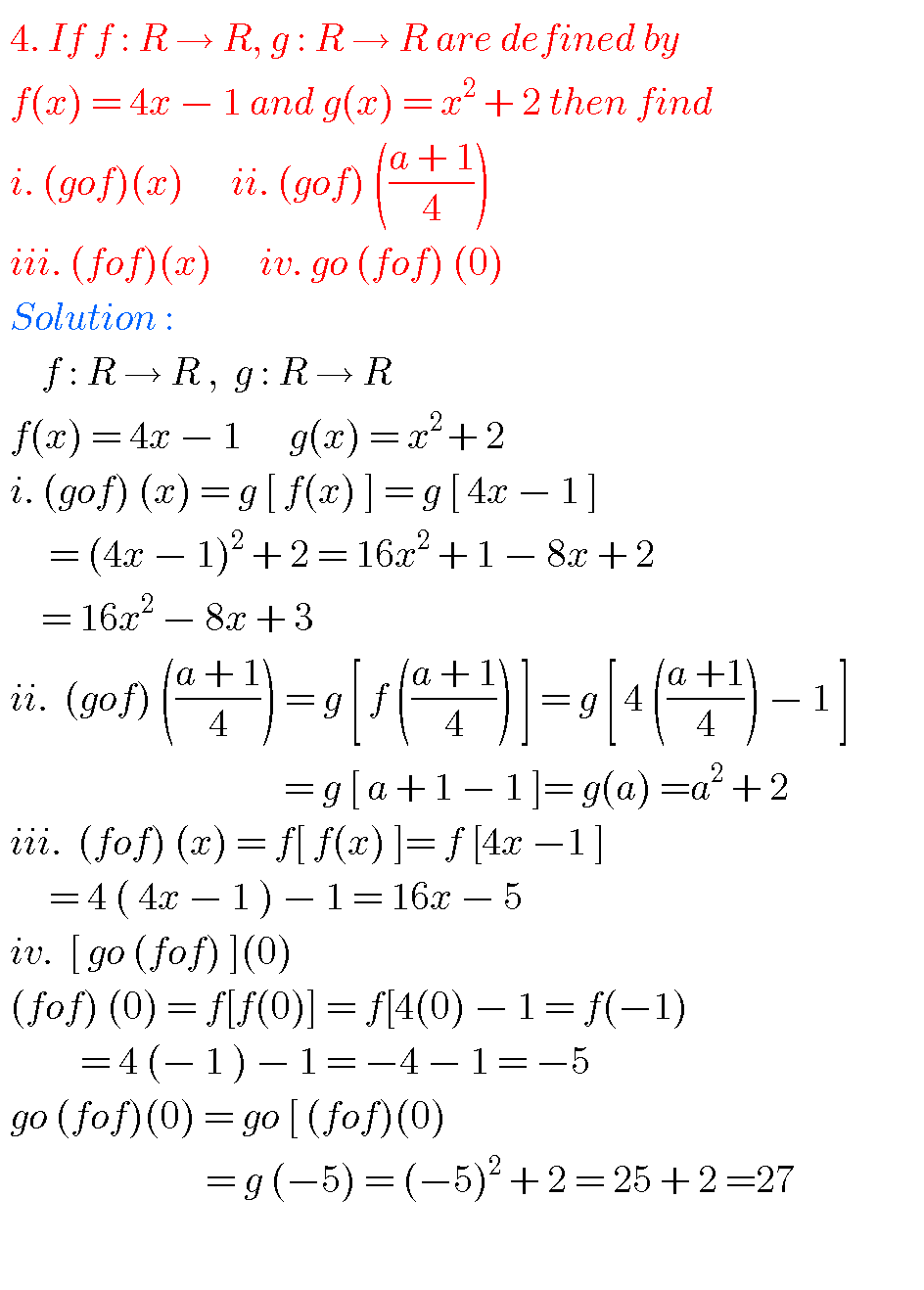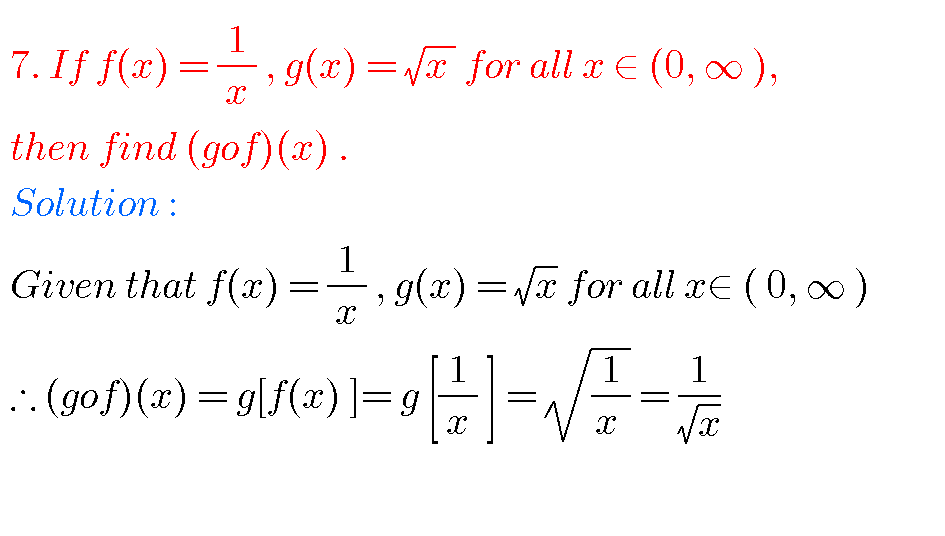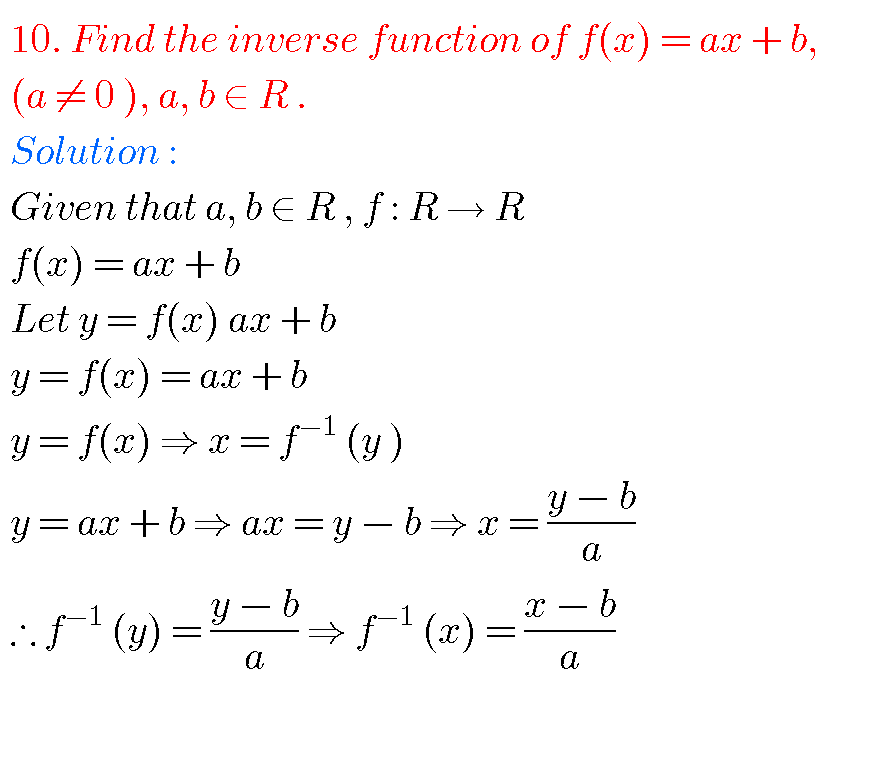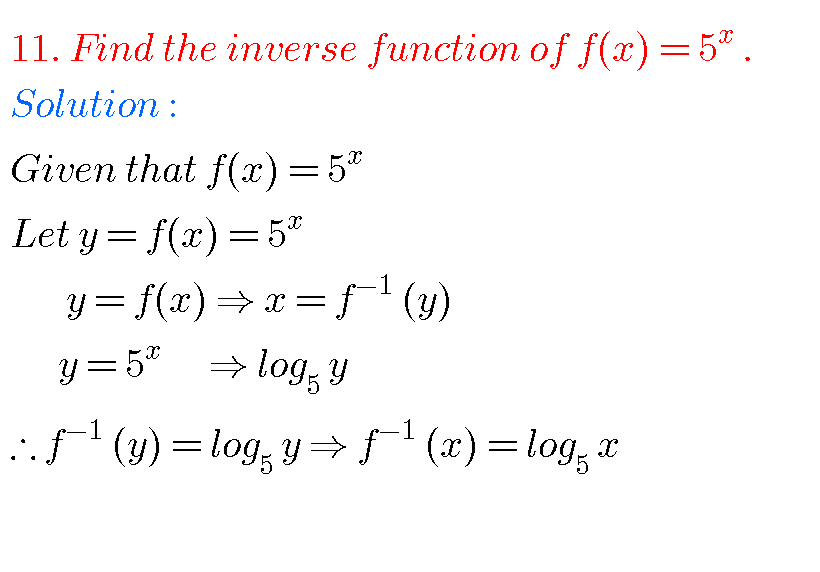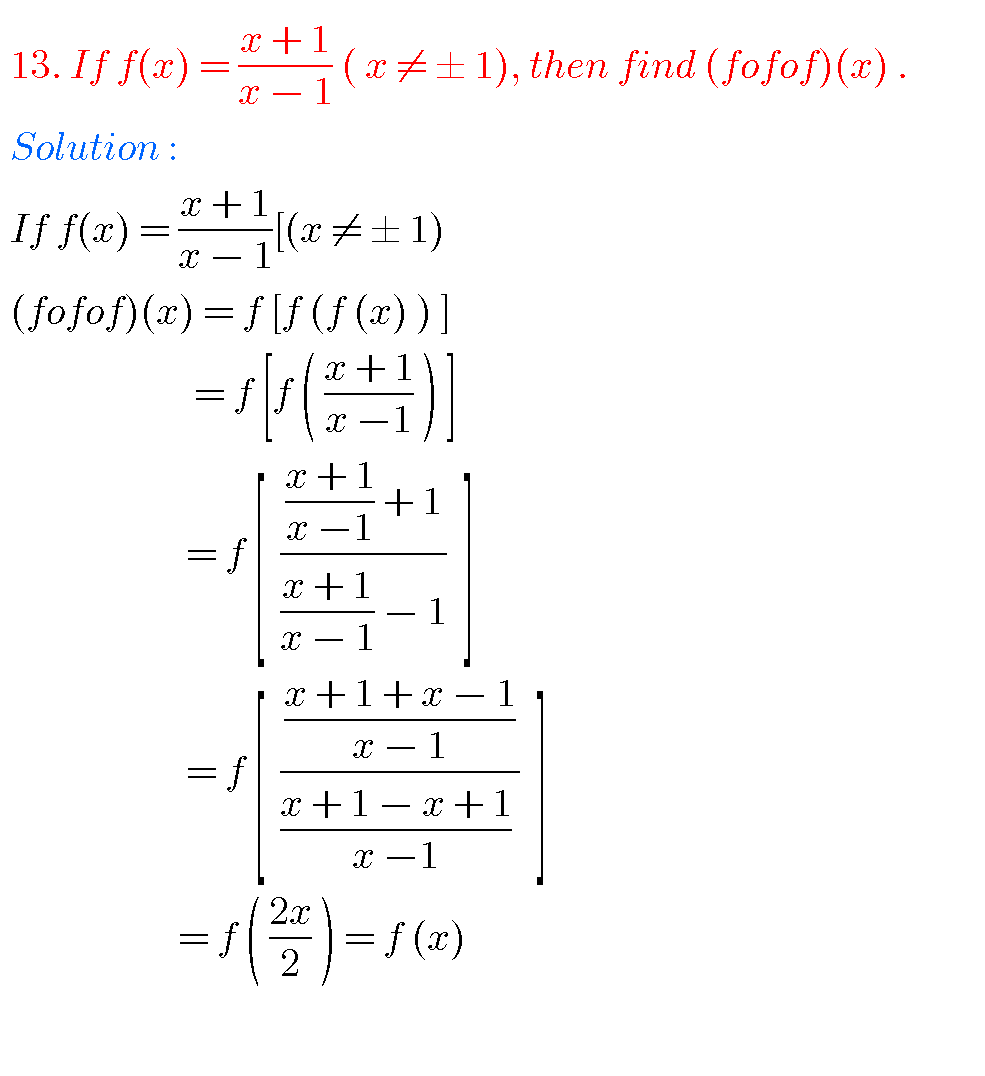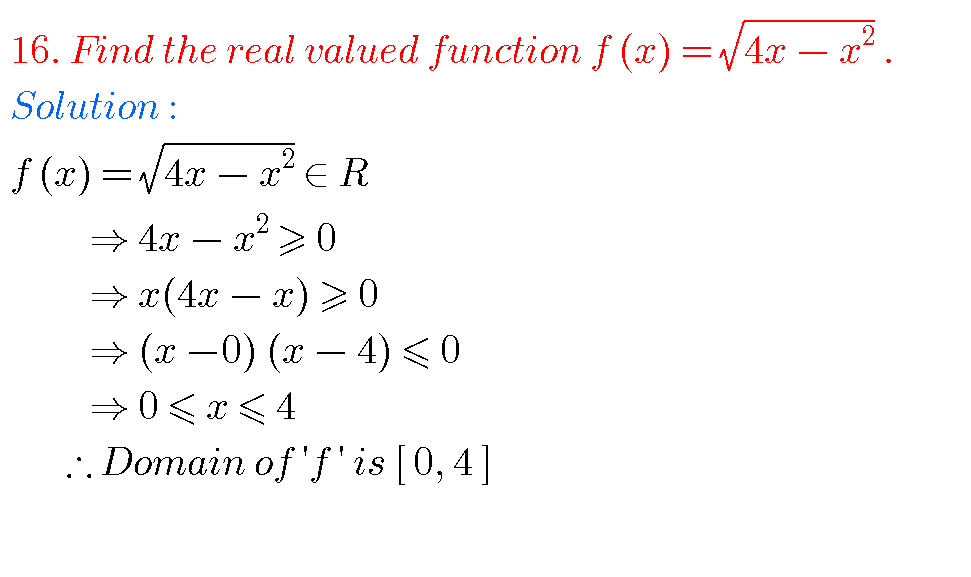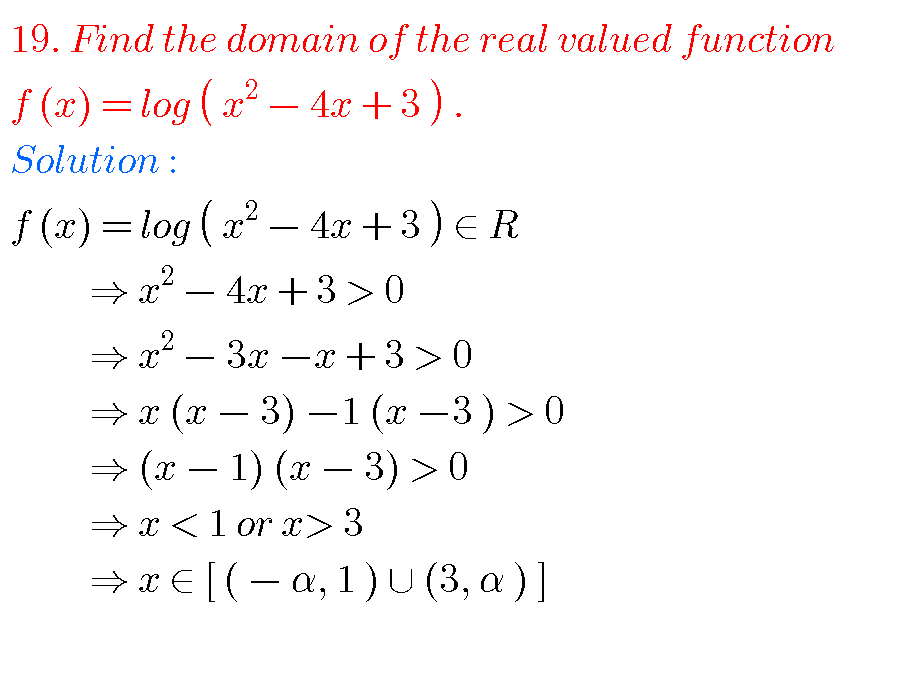# SOLUTIONS OF FUNCTIONS INTERMEDIATE FIRST YEAR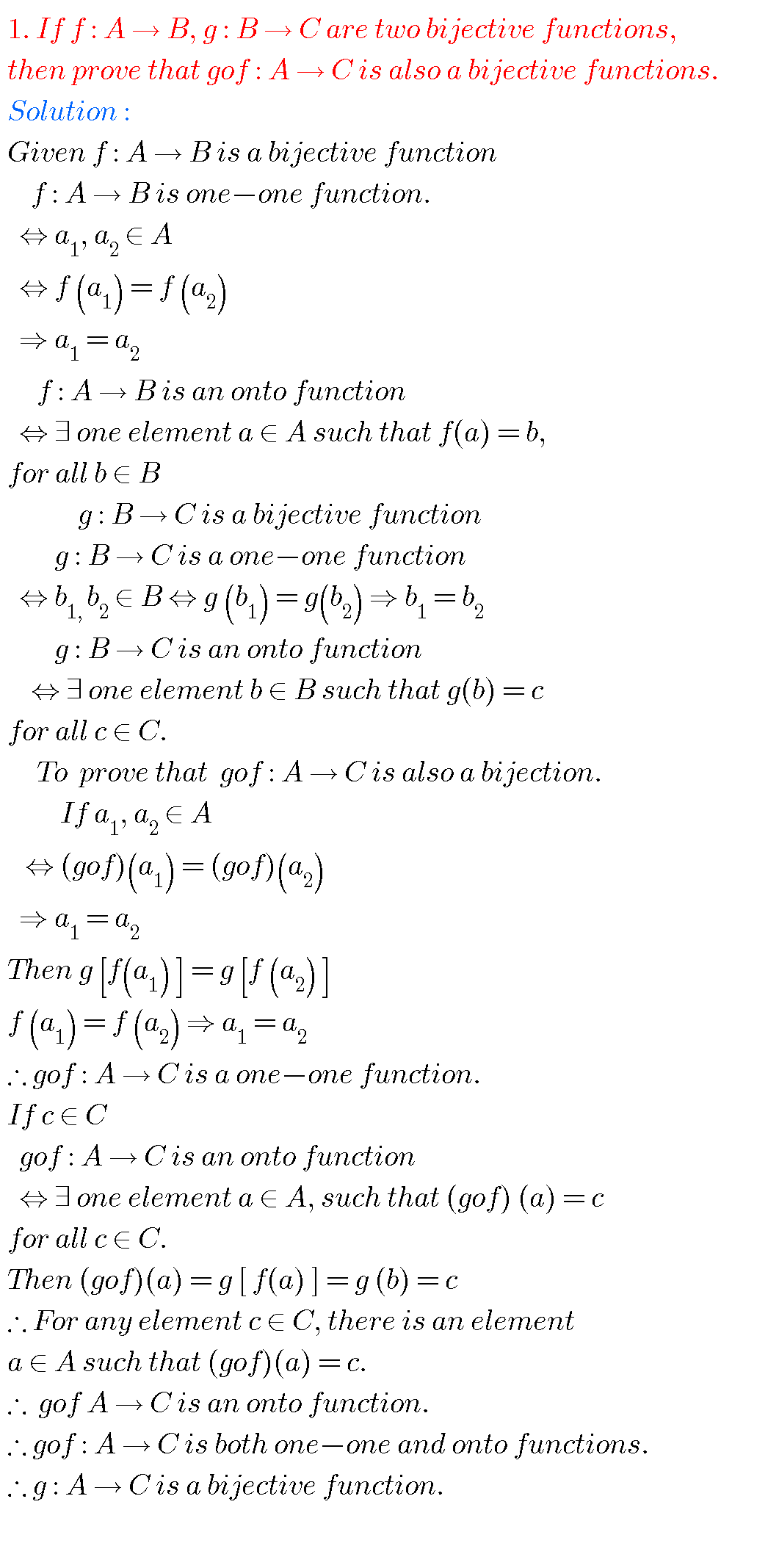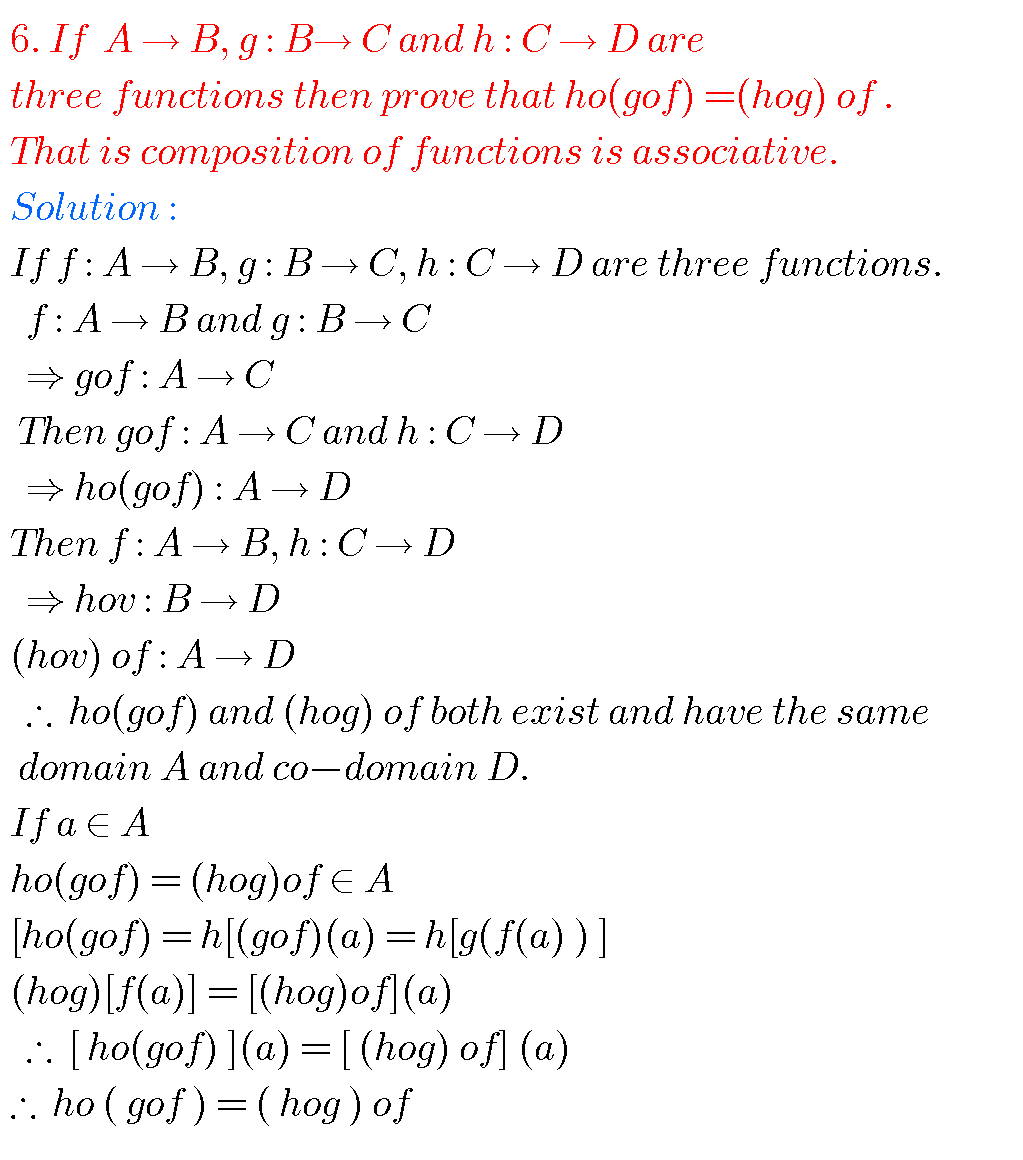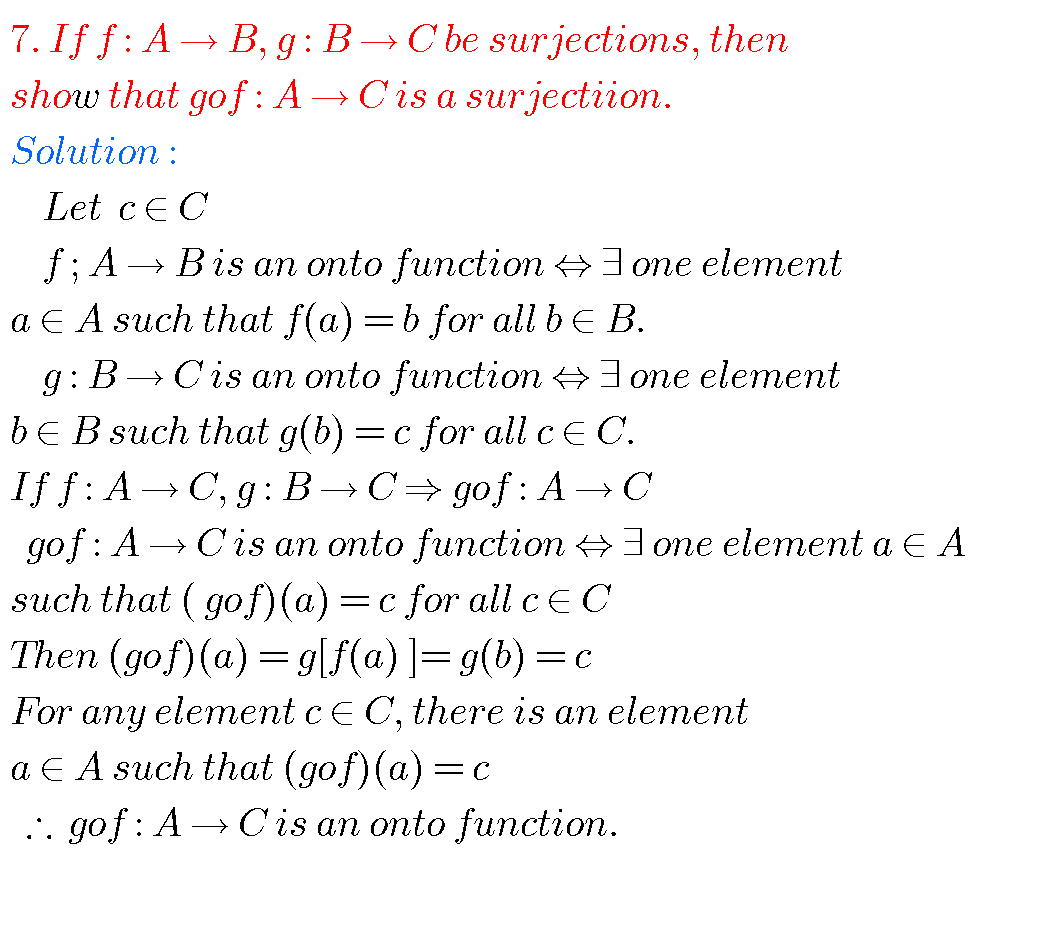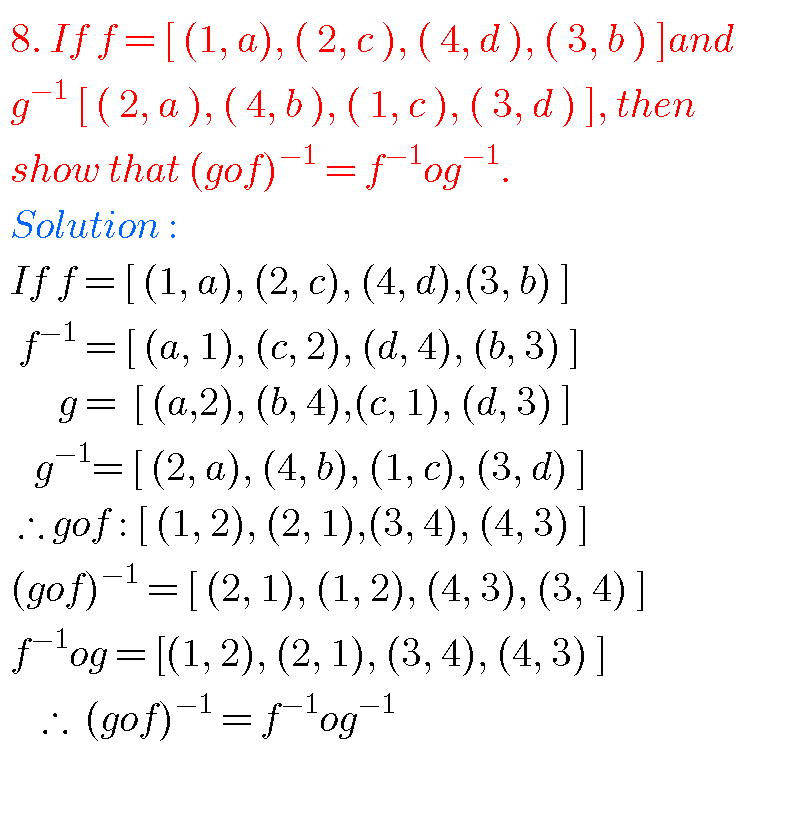### FUNCTIONS SOLUTIONS INTERMEDIATE

Note : Observe the solutions of functions and try them in your own methods.

### 1 thought on “Functions,Intermediate,Solutions”

1.Tq